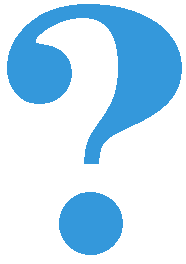# Obeleži sve kategorije koje odgovaraju problemu

### Još detalja - opišite nam problem

Uspešno ste prijavili problem!
Status problema i sve dodatne informacije možete pratiti klikom na link.
Nažalost nismo trenutno u mogućnosti da obradimo vaš zahtev.
Molimo vas da pokušate kasnije.

# Additional instructions to a function¶

We mentioned that there are several ways to write functions in Python, and that the functions we have written and used so far have the simplest form. Such functions are, for example, move(), turn_left(), turn_right(), pick_ball() and drop_ball() from the Karel Library, as well as the functions back(), take_at_neighboring_square() and go_to_neighboring_nonempty_square(), which we wrote ourselves. All these functions perform a specific job, always in the same way.

Functions can be written so that in different executions they do not always do the exact same thing, but they perform a little more general task. When we call such functions, we tell them exactly how we want them to do their task. For example, a function that would move Karel for a certain number of squares forward or backward could often be very useful. For this function, we want to specify the request when calling it - how many squares Karel should move and on which side.

## Functions with parameters¶

Additional information that we give to a function is written between the brackets after the function name in the first row of its definition. Between brackets we can specify one value, or multiple values separated by commas. These values are called arguments or parameters to a function. The words “arguments” and “parameters” are synonyms in programming and we will use them equally.

The function that moves Karel certain number of squares forward or backward, could be named go and it could have one integer parameter. If this parameter is positive, Karel would move that many squares forward, and if it is negative, Karel would go a corresponding (opposite) number of squares back. For example, the call go(5) would mean “go 5 squares forward”, while go(-2) would mean “go 2 squares back”. Here’s how we can write such a function:

This function can simplify many programs that are supposed to tell Karel to move several times along one path in both directions. Here is an example.

### Perform specified displacements¶

####Karel is located on the starting square of a path of a sufficient length, and should carry out the following displacements of balls:

• 3 balls from the square 3 to the square 4

• 4 balls from the square 5 to the square 1

In solving this task we will use the described function go. In order to further simplify the solution, we can also introduce the displace function, which displaces the specified number of balls for a specified number of squares forward or backward. This description shows that the displace function should have two arguments.

In order to make the purpose of each parameter clearer, we will give them names that describe their role:

The displace function uses the previously written function go. Calling a function from another function like this can go in depth as far as we need. It is only important that each function is defined before it is called for execution.

Now that we have these two functions available, solving the starting task is very easy:

(Karel_functions__displace_balls_eng)

### Take a given number of balls¶

####Write the function take_up_to(n), which tells Karel to take the maximum of n balls from the square which he stands on. More precisely, if there are n or more balls at the square, Karel takes n of them, and if there are fewer balls, Karel takes as many as he can.

Karel, who is on the first square, should take up to 4 balls from the second square, then up to 2 balls from the third square, and up to 3 balls from the fourth square, and then bring all the collected balls to the first square. Of course, the take_up_to(n) function, written in the first part of the task, should be used for this purpose.

(Karel_functions__take_balls_up_to_eng)

### Driving according to instructions¶

####The functions face_left_at_intersection() and go_left(n) are given.

• Function face_left_at_intersection() positions Karel to face the first street he comes across on the left side. In the execution of this function, Karel goes forward until he encounters a square where he can go left, but he does not actually go left, he remains on the crossroad instead, turned to the left. If Karel can go left before the function call, he will not move from its square during the execution of this function, but will only turn to the left;

• The function go_left(n) moves Karel one square to the n-th street on the left. If Karel is already in the crossroads, the street to the left of him is counted as the first;

Write similar functions face_left_at_intersection() and go_right(n) using given functions as a model.

Write a program that (using given and written functions) leads Karel to the third street to the left, then the second to the right, and at the end, the second to the left. Karel should reach the end of that street and take the only ball on the table.